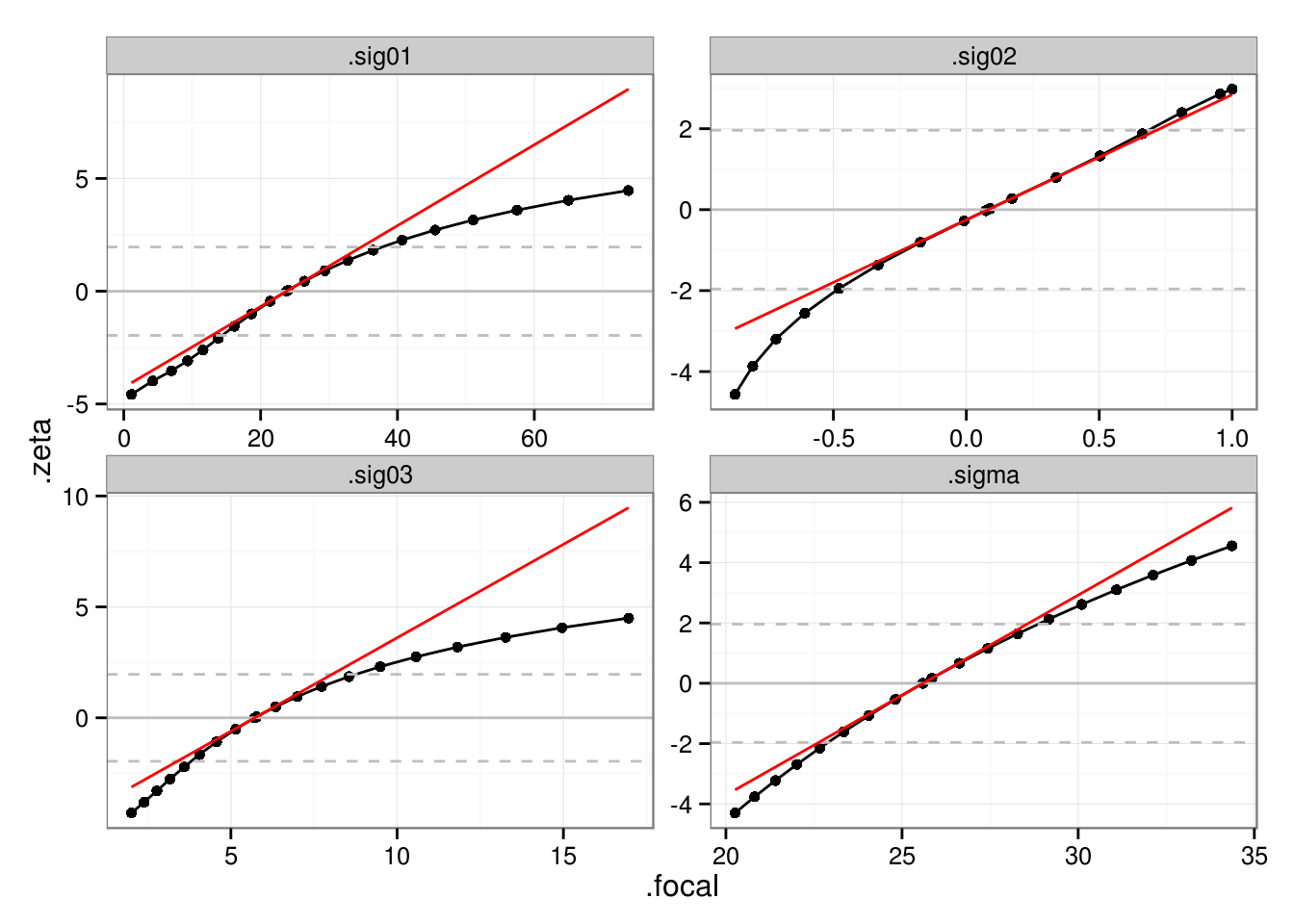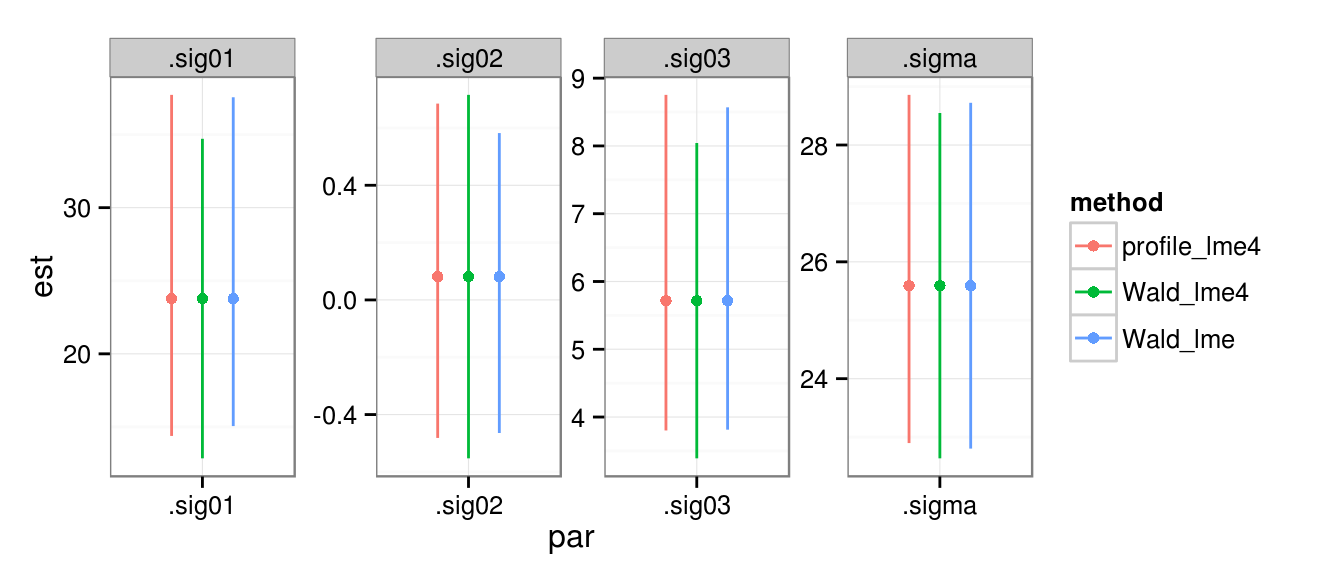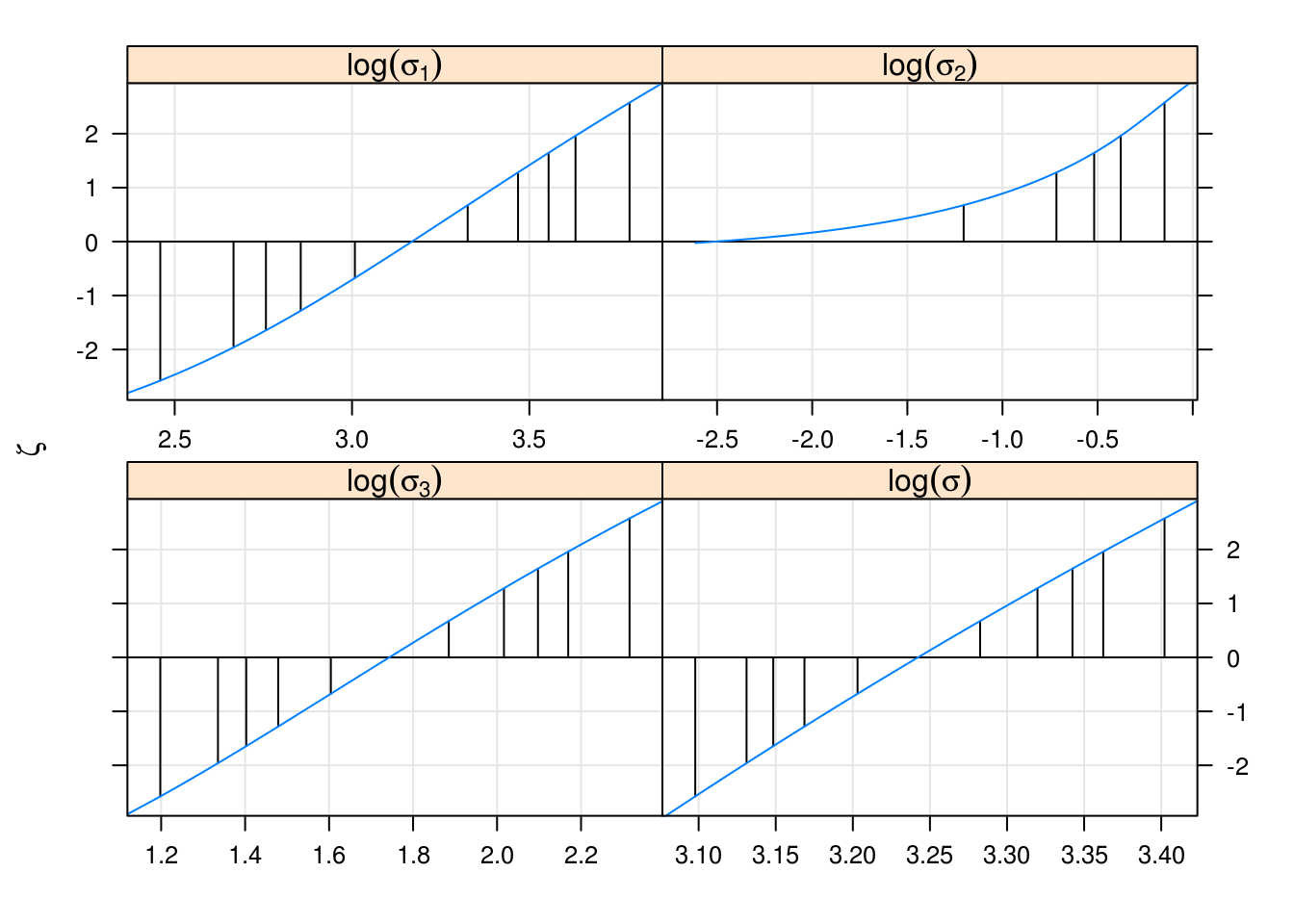## Calculating the variance-covariance matrix of variance-covariance parameters

(Note: the generic example below requires the very latest (post-17 Sep 2014) development version of lme4, which adds the order argument to as.data.frame.VarCorr.merMod)

One relatively frequent request from lme4 users is for the capability to compute the variance-covariance matrix of the variance parameters themselves. In the nlme package this was (implicitly) available via the intervals() function i the nlme package (which returned Wald confidence intervals); lme4 has switched to providing profile and parametric bootstrap, and not Wald confidence intervals, because the Wald intervals on variance-covariance parameters are often really, really bad.

Nevertheless, there are sometimes reasons that one wants to get the asymptotic (Wald) variance-covariance matrix of the variance-covariance parameters themselves. This document shows how to do that for ML estimates, and discusses the issues more generally (so that hopefully a similar solution for the REML estimates will also be feasible soon).

The basic problem is lme4’s deviance function uses a scaled Cholesky-factor parameterization: it’s easy enough to get an approximate Hessian of these ($$\theta$$) parameters by finite differencing, but annoying to convert them to the $$\sigma$$ or $$\sigma^2$$ scale. However, for ML estimates we can use an internal function (devfun2) that is parameterized on the $$\sigma$$ scale. (To get asymptotic variance-covariance estimates for REML estimates we will either need to adapt devfun2 to the REML scale, or figure out a more direct scale conversion: see notes below.

note: devfun2, which is an internal lme4 function, may be a little bit fragile when used inside functions (hence the vcov.VarCorr.merMod function below inherits that fragility)

### Preliminaries

Fit the good old sleepstudy model:

library(lme4)
fm1 <- lmer(Reaction ~ Days + (Days | Subject), sleepstudy)

For comparison with the likelihood profile (which uses ML rather than REML) we also fit the ML equivalent:

fm1.ML <- refitML(fm1)

Also fit with lme so that we can compare the results of intervals():

library(nlme)
fm1.lme <- lme(Reaction ~ Days, random = ~Days| Subject, sleepstudy)
fm1.lme.ML <- update(fm1.lme,method="ML")

### Finite differences on the $$\sigma$$ scale

The easiest way to do what we want is to use the internal devfun2() function, which is used within profile — it is essentially the same as the basic devfun(), but it uses a standard deviation/correlation parameterization (and adds the residual standard deviation as a parameter) rather than a Cholesky parameterization:

dd.ML <- lme4:::devfun2(fm1.ML,useSc=TRUE,signames=FALSE)

At the moment I think devfun2() cannot be used for REML fits (as it is designed for computing likelihood profiles, that wouldn’t make much sense).

The other way to do this would be to compute the Hessian for the regular deviance function, then do the requisite calculus and linear algebra on the function $${\cal F}$$ that translates from the Cholesky to the stddev/corr parameterization to convert the Hessian to the new parameterization. (Since we probably want to develop that machinery anyway for other transformations, such as log-transformations, that aren’t covered by devfun2, we might do better to start there.)

Retrieve the standard deviation/correlation parameters, in the correct order:

vv <- as.data.frame(VarCorr(fm1.ML)) ## need ML estimates!
## name for consistency with profile object below
vnames <- c(sprintf(".sig%02d",1:3),".sigma")
pars <- setNames(vv[c(1,3,2,4),"sdcor"],vnames)

Compute second derivatives (the factor of 2 converts from the deviance to the log-likelihood scale):

library(numDeriv)
hh1 <- hessian(dd.ML,pars)
vv2 <- 2*solve(hh1)

### Results and comparisons

Now that we have the Wald variance-covariance matrix, I’m going to cook up a little bit of structure that will let us use confint.default to compute the Wald intervals:

dimnames(vv2) <- list(vnames,vnames)
vhack <- list(coefficients=pars,vcov=vv2)
vcov.default <- function(object,...) object$vcov (wci <- confint.default(vhack)) ## 2.5 % 97.5 % ## .sig01 12.849120 34.7120134 ## .sig02 -0.552733 0.7153734 ## .sig03 3.390218 8.0434502 ## .sigma 22.636166 28.5474654 These are close, although not precisely the same as, the Wald intervals produced by lme. (Note that the order differs — the lme4 stuff is using “lower-triangular” order, so that the correlation (.sig02) comes between the two standard deviation estimates, while lme (and the as.data.frame.VarCorr.merMod method from lme4) puts the correlations last by default – but see updates/generic function below.) (lmeint <- intervals(fm1.lme.ML,which="var-cov")) ## Approximate 95% confidence intervals ## ## Random Effects: ## Level: Subject ## lower est. upper ## sd((Intercept)) 15.0589630 23.7803757 37.5528031 ## sd(Days) 3.8137863 5.7168073 8.5694065 ## cor((Intercept),Days) -0.4643561 0.0813321 0.5822599 ## ## Within-group standard error: ## lower est. upper ## 22.80274 25.59184 28.72209 #### Comparison with likelihood profile Compute the likelihood profile: pp <- profile(fm1,which="theta_") The linear approximation to the profile for a focal parameter $$x$$ should be $$\zeta \approx (x-\hat x)/\sigma_x$$, where $$\hat x$$ is the MLE and $$\sigma_x$$ is the Wald standard deviation:In this particular example, there’s not a huge divergence from linearity over the range defined by the 95% confidence intervals (dashed horizontal lines) … as confirmed by the confidence intervals. Once we compute the second derivatives on the log scale, we can similarly compare them with logProf(pp). #### Comparison of CIsIn fact, we know from internal inspection that the lme Wald intervals are calculated on a different scale, i.e. the log-$$\sigma$$ scale, which are even closer to the profile CIs … i.e. these curves (at least those for $$\log(\sigma_3)$$, $$\log(\sigma)$$) are closer to linear than those shown above: lattice::xyplot(logProf(pp),as.table=TRUE)### Generalizing It’s nice to make this into a generic method for VarCorr.merMod objects; the only annoyance is that we also need to pass the fitted object. vcov.VarCorr.merMod <- function(object,fit,...) { if (isREML(fit)) { warning("refitting model with ML") fit <- refitML(fit) } if (!require("numDeriv")) stop("numDeriv package required") useSc <- attr(object,"useSc") dd <- lme4:::devfun2(fit,useSc=useSc,signames=FALSE) vdd <- as.data.frame(object,order="lower.tri") pars <- vdd[,"sdcor"] npar0 <- length(pars) if (isGLMM(fit)) { pars <- c(pars,fixef(fit)) } hh1 <- hessian(dd,pars) vv2 <- 2*solve(hh1) if (isGLMM(fit)) { vv2 <- vv2[1:npar0,1:npar0,drop=FALSE] } nms <- apply(vdd[,1:3],1, function(x) paste(na.omit(x),collapse=".")) dimnames(vv2) <- list(nms,nms) return(vv2) } Test: all.equal(unname(vhack$vcov),unname(vcov(VarCorr(fm1.ML),fm1.ML)))
##  TRUE

Try it on a GLMM example:

gm1 <- glmer(cbind(incidence, size - incidence) ~ period + (1 | herd),
data = cbpp, family = binomial)
vcov(VarCorr(gm1),gm1)
##                  herd.(Intercept)
## herd.(Intercept)       0.03152581

### Continuing: transformations via chain rule

If we want the derivatives on a different scale (e.g. the derivatives of the deviance with respect to $$\log(x)$$, or more generally the derivatives of the deviance with respect to any monotonic transformation of $$x$$), in principle we just need to use the chain rule. I am currently getting terribly, embarrassingly muddled in trying to figure out how to apply the chain rule properly in this case.

• given the deviance function $$D(x)$$ and the estimated derivative at the MLE $$\hat D(x)$$, figure out how to get the derivative based on the chain rule – this should just be zero, because monotonic transformation doesn’t change the critical points.
• figure out how to get the second derivative of $$D(g(x))$$ via the chain rule (I think this should really boil down to something embarrassingly simple like $$1/g'(x)^2$$, based on a scale-transformation argument, but it would be nice to see it derived properly).
• figure out how to transform the full Hessian (second derivative matrix) for a set of transformations $$F=\{f_1(x_1),f_2(x_2),f_3(x_3), \ldots}$$ (e.g. if we want to log-transform standard deviations and logit-transform correlations).
• figure out the transformation/chain rule from the $$\theta$$ scale to arbitrary scales (then we wouldn’t need devfun2 any more …)

More generally, if we have a multidimensional parameter transformation $$F$$ we can (approximately) transform variances via the rule given in the “standard errors” section of this document on hazards and odds ratios

Can I get the second derivative via chain rule (can’t remember any more why I wanted this …)? We’re looking for $$\frac{\partial^2 f(g(x))}{\partial x^2}$$ (or $$\frac{\partial^2 (f \circ g)(x)}{\partial x^2}$$ may be easier notation in this case).

I should have been able to figure this out myself, but looking at Faà di Bruno’s formula:

$\frac{\partial^n}{\partial x_1 \dots \partial x_n} = \sum_{\pi \in \Pi} f^{(|\pi|}(y) \cdot \prod_{B \in \pi} \frac{\partial^{|B|}y}{\prod_{j \in B} \partial x_j}$

For $$n=2$$ the partitions are just $$\{\{1\},\{2\}\}$$ and $$\{\{1,2\}\}$$ $\frac{\partial^2 f(y)}{\partial x_1 x_2} = f'(y) \frac{\partial^2 y}{\partial x_1 x_2} + f''(y) \frac{\partial y}{\partial x_1} \frac{\partial y}{\partial x_2}$

The derivative of a matrix cross-product $$X X^T$$ should be fairly straightforward (I think?); e.g. for a two-by-two crossproduct works out as $$(\lambda \lambda^T)' = \lambda' \lambda^T + \lambda (\lambda^T)'$$, where differentiation is element-by-element on the RHS.